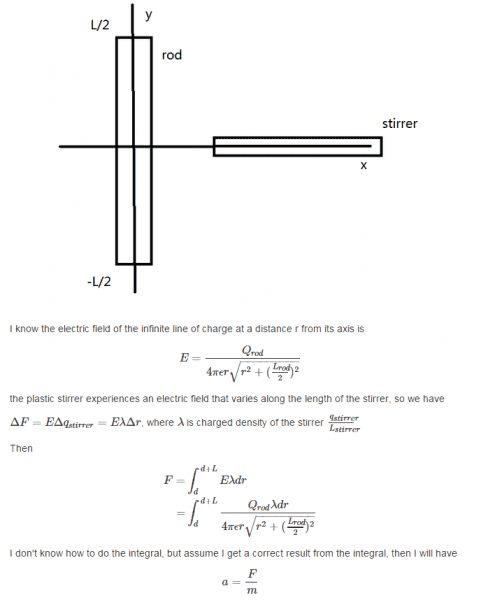# Acceleration of a stirrer

## Homework Statement

Question: There is a fixed 55 cm long rod (vertical) that is charged uniformly up to +137 nC, Place a 0.5 g plastic 6 cm long stirrer (horizontal) that is charge to +93 nC, 2.3 cm away from the rod

Calculate the Electric force and acceleration on the stirrer .

## Homework Equations

E=Q/4piε r√r^2+(l/2)^2
F=qE=ma

## The Attempt at a Solution[/B]

haruspex
Homework Helper
Gold Member
2020 Award
I guess you meant 'finite line', not infinite.
Try a trig substitution for r. What is suggested by the r2+constant part?

I guess you meant 'finite line', not infinite.
Try a trig substitution for r. What is suggested by the r2+constant part?
Let r =sqrt (l/2) tantheta?

haruspex
Homework Helper
Gold Member
2020 Award
Let r =sqrt (l/2) tantheta?
Looks good.

Looks good.
Is that reasonable to get a=0.003m/s^2 ?

haruspex